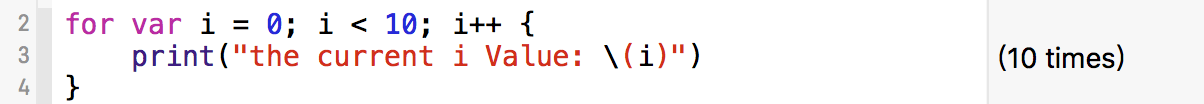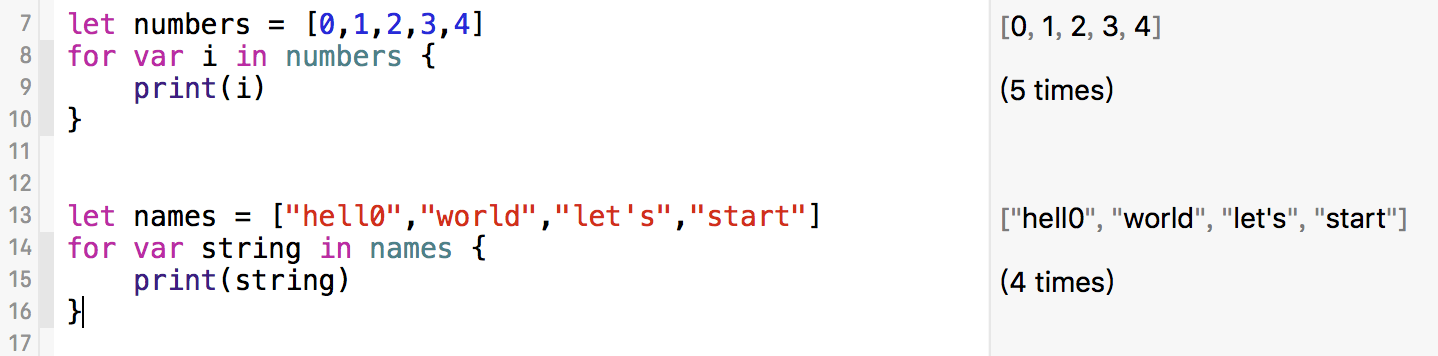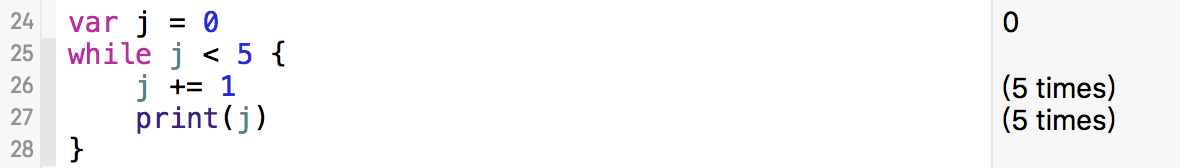# 普通for循环

``````//传统写法
for var i = 0; i < 10; i++ {
print("the current i Value: \(i)")
}

//swift3.0推荐写法
for i in 0 ..< 10 {
print("the current i Value: \(i)")
}
``````# forin循环

``````let numbers = [0,1,2,3,4]
for var i in numbers {
print(i)
}

let names = ["hell0","world","let's","start"]
for var string in names {
print(string)
}
``````# while循环

``````var j = 0
while j < 5 {
j += 1
print(j)
}
``````# 代码下载

https://github.com/99ios/SwiftBasic